## Projection onto a SubspaceFigure 1

Let S be a nontrivial subspace of a vector space V and assume that v is a vector in V that does not lie in S. Then the vector v can be uniquely written as a sum, v S + v S , where v S is parallel to S and v S is orthogonal to S; see Figure .

The vector v S , which actually lies in S, is called the projection of v onto S, also denoted proj S v. If v 1, v 2, …, v r form an orthogonal basis for S, then the projection of v onto S is the sum of the projections of v onto the individual basis vectors, a fact that depends critically on the basis vectors being orthogonal:Figure shows geometrically why this formula is true in the case of a 2‐dimensional subspace S in R 3.Figure 2

Example 1: Let S be the 2‐dimensional subspace of R 3 spanned by the orthogonal vectors v 1 = (1, 2, 1) and v 2 = (1, −1, 1). Write the vector v = (−2, 2, 2) as the sum of a vector in S and a vector orthogonal to S.

From (*), the projection of v onto S is the vectorTherefore, v = v S where v S = (0, 2, 0) andThat v S = (−2, 0, 2) truly is orthogonal to S is proved by noting that it is orthogonal to both v 1 and v 2:In summary, then, the unique representation of the vector v as the sum of a vector in S and a vector orthogonal to S reads as follows:See Figure .Figure 3

Example 2: Let S be a subspace of a Euclidean vector space V. The collection of all vectors in V that are orthogonal to every vector in S is called the orthogonal complement of S:( S is read “S perp.”) Show that S is also a subspace of V.

Proof. First, note that S is nonempty, since 0S . In order to prove that S is a subspace, closure under vector addition and scalar multiplication must be established. Let v 1 and v 2 be vectors in S ; since v 1 · s = v 2 · s = 0 for every vector s in Sproving that v 1 + v 2S . Therefore, S is closed under vector addition. Finally, if k is a scalar, then for any v in S , ( k v) · s = k( v · s) = k(0) = 0 for every vector s in S, which shows that S is also closed under scalar multiplication. This completes the proof.

Example 3: Find the orthogonal complement of the x−y plane in R 3.

At first glance, it might seem that the x−z plane is the orthogonal complement of the x−y plane, just as a wall is perpendicular to the floor. However, not every vector in the x−z plane is orthogonal to every vector in the x−y plane: for example, the vector v = (1, 0, 1) in the x−z plane is not orthogonal to the vector w = (1, 1, 0) in the x−y plane, since v · w = 1 ≠ 0. See Figure . The vectors that are orthogonal to every vector in the x−y plane are only those along the z axis; this is the orthogonal complement in R 3 of the x−y plane. In fact, it can be shown that if S is a k‐dimensional subspace of R n , then dim S = n − k; thus, dim S + dim S = n, the dimension of the entire space. Since the x−y plane is a 2‐dimensional subspace of R 3, its orthogonal complement in R 3 must have dimension 3 − 2 = 1. This result would remove the x−z plane, which is 2‐dimensional, from consideration as the orthogonal complement of the x−y plane.Figure 4

Example 4: Let P be the subspace of R 3 specified by the equation 2 x + y = 2 z = 0. Find the distance between P and the point q = (3, 2, 1).

The subspace P is clearly a plane in R 3, and q is a point that does not lie in P. From Figure , it is clear that the distance from q to P is the length of the component of q orthogonal to P.Figure 5

One way to find the orthogonal component q P is to find an orthogonal basis for P, use these vectors to project the vector q onto P, and then form the difference q − proj P q to obtain q P . A simpler method here is to project q onto a vector that is known to be orthogonal to P. Since the coefficients of x, y, and z in the equation of the plane provide the components of a normal vector to P, n = (2, 1, −2) is orthogonal to P. Now, sincethe distance between P and the point q is 2.

The Gram‐Schmidt orthogonalization algorithm. The advantage of an orthonormal basis is clear. The components of a vector relative to an orthonormal basis are very easy to determine: A simple dot product calculation is all that is required. The question is, how do you obtain such a basis? In particular, if B is a basis for a vector space V, how can you transform B into an orthonormal basis for V? The process of projecting a vector v onto a subspace S—then forming the difference v − proj S v to obtain a vector, v S , orthogonal to S—is the key to the algorithm.

Example 5: Transform the basis B = { v 1 = (4, 2), v 2 = (1, 2)} for R 2 into an orthonormal one.

The first step is to keep v 1; it will be normalized later. The second step is to project v 2 onto the subspace spanned by v 1 and then form the difference v 2proj v1 v 2 = v ⊥1 Since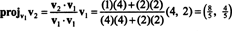the vector component of v 2 orthogonal to v 1 is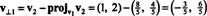as illustrated in Figure .Figure 6

The vectors v 1 and v ⊥1 are now normalized:Thus, the basis B = { v 1 = (4, 2), v 2 = (1, 2)} is transformed into the orthonormal basis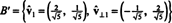shown in Figure .Figure 7

The preceding example illustrates the Gram‐Schmidt orthogonalization algorithm for a basis B consisting of two vectors. It is important to understand that this process not only produces an orthogonal basis B′ for the space, but also preserves the subspaces. That is, the subspace spanned by the first vector in B′ is the same as the subspace spanned by the first vector in B′ and the space spanned by the two vectors in B′ is the same as the subspace spanned by the two vectors in B.

In general, the Gram‐Schmidt orthogonalization algorithm, which transforms a basis, B = { v 1, v 2,…, v r }, for a vector space V into an orthogonal basis, B′ { w 1, w 2,…, w r }, for V—while preserving the subspaces along the way—proceeds as follows:

Step 1. Set w 1 equal to v 1

Step 2. Project v 2 onto S 1, the space spanned by w 1; then, form the difference v 2proj S 1 v 2 This is w 2.

Step 3. Project v 3 onto S 2, the space spanned by w 1 and w 2; then, form the difference v 3proj S 2 v 3. This is w 3.

Step i. Project v i onto S i −1, the space spanned by w 1, …, w i−1 ; then, form the difference v i proj S i−1 v i . This is w i .

This process continues until Step r, when w r is formed, and the orthogonal basis is complete. If an orthonormal basis is desired, normalize each of the vectors w i .

Example 6: Let H be the 3‐dimensional subspace of R 4 with basisFind an orthogonal basis for H and then—by normalizing these vectors—an orthonormal basis for H. What are the components of the vector x = (1, 1, −1, 1) relative to this orthonormal basis? What happens if you attempt to find the componets of the vector y = (1, 1, 1, 1) relative to the orthonormal basis?

The first step is to set w 1 equal to v 1. The second step is to project v 2 onto the subspace spanned by w 1 and then form the difference v 2proj W1 v 2 = W 2. Sincethe vector component of v 2 orthogonal to w 1 isNow, for the last step: Project v 3 onto the subspace S 2 spanned by w 1 and w 2 (which is the same as the subspace spanned by v 1 and v 2) and form the difference v 3proj S 2 v 3 to give the vector, w 3, orthogonal to this subspace. Sinceand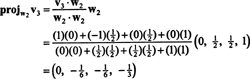and { w 1, w 2} is an orthogonal basis for S 2, the projection of v 3 onto S 2 isThis gives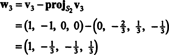Therefore, the Gram‐Schmidt process produces from B the following orthogonal basis for HYou may verify that these vectors are indeed orthogonal by checking that w 1 · w 2 = w 1 · w 3 = w 2 · w 3 = 0 and that the subspaces are preserved along the way: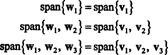An orthonormal basis for H is obtained by normalizing the vectors w 1, w 2, and w 3Relative to the orthonormal basis B′′ = { ŵ 1, ŵ 2, ŵ 3}, the vector x = (1, 1, −1, 1) has componentsThese calculations imply thata result that is easily verified.

If the components of y = (1, 1, 1, 1) relative to this basis are desired, you might proceed exactly as above, findingThese calculations seem to imply thatThe problem, however, is that this equation is not true, as the following calculation shows:What went wrong? The problem is that the vector y is not in H, so no linear combination of the vectors in any basis for H can give y. The linear combinationgives only the projection of y onto H.

Example 7: If the rows of a matrix form an orthonormal basis for R n , then the matrix is said to be orthogonal. (The term orthonormal would have been better, but the terminology is now too well established.) If A is an orthogonal matrix, show that A −1 = A T.

Let B = { 1, 2, …, n } be an orthonormal basis for R n and consider the matrix A whose rows are these basis vectors: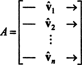The matrix A T has these basis vectors as its columns:Since the vectors 1, 2, …, n are orthonormal,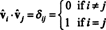Now, because the ( i, j) entry of the product AA T is the dot product of row i in A and column j in A T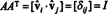Thus, A −1 = A T. [In fact, the statement A −1 = A T is sometimes taken as the definition of an orthogonal matrix (from which it is then shown that the rows of A form an orthonormal basis for R n ).]

An additional fact now follows easily. Assume that A is orthogonal, so A −1 = A T. Taking the inverse of both sides of this equation giveswhich implies that A T is orthogonal (because its transpose equals its inverse). The conclusionmeans that if the rows of a matrix form an orthonormal basis for R n , then so do the columns.